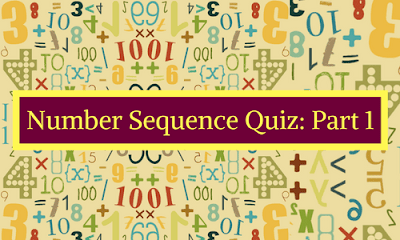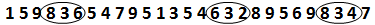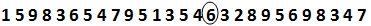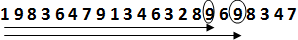New Students Offer - Use Code HELLO

# Number Sequence Quiz: Part 1Directions (Q.1-5): Study the following number sequence and answer the question given below:

1 5 9 8 3 6 5 4 7 9 5 1 3 5 4 6 3 2 8 9 5 6 9 8 3 4 7

Q.1 How many such 3s are in given number sequence that are immediately preceding by an even number but not followed by a number that is not divisible by 2?
A. None
B. 4
C. 2
D. 3
E. 5

Q.2 Which of the following is the fifth to the left of the seventh from the right end of the given arrangement?
A. 6
B. 4
C. 2
D. 3
E. 7

Q.3 If all the 5s are deleted from the given number sequence then what will be the position of 9 that is preceded and followed by an even number from the left end?
A. 16th
B. 19th
C. 18th
D. A and C
E. A and B

Q.4 How many pairs of successive numbers have the difference of 3 each?
A. 3
B. 5
C. 4
D. 2
E. None

Q.5 How many numbers are there which are divisible by 2 or 3 and followed by a number that is divisible by 2 in the given number sequence?
A. 5
B. 15
C. 10
D. 7
E. 12

#### 1. Ans. D.

Solution:#### 2. Ans. A.

Solution:#### 3. Ans.E

Solution:#### 4. Ans. B

Solution:#### 5. Ans. D.

Solution: